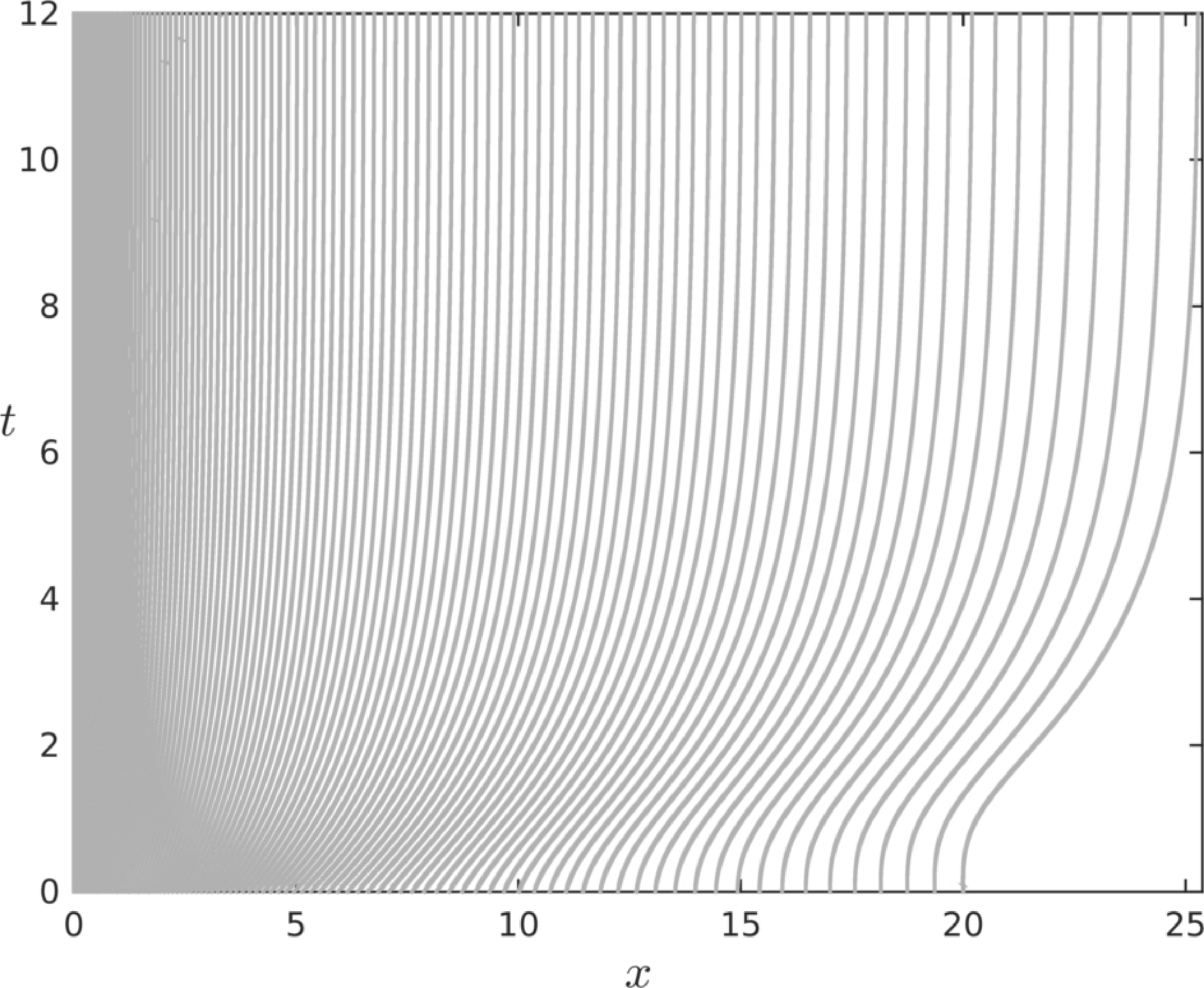Preprint Article Version 1 Preserved in Portico This version is not peer-reviewed

# Numerical Simulation of Feller's Diffusion Equation

Version 1 : Received: 12 September 2019 / Approved: 12 September 2019 / Online: 12 September 2019 (15:36:44 CEST)

A peer-reviewed article of this Preprint also exists.

Dutykh, D. Numerical Simulation of Feller’s Diffusion Equation. Mathematics 2019, 7, 1067. Dutykh, D. Numerical Simulation of Feller’s Diffusion Equation. Mathematics 2019, 7, 1067.

Journal reference: Mathematics 2019, 7, 1067
DOI: 10.3390/math7111067

## Abstract

This article is devoted to Feller's diffusion equation which arises naturally in probabilities and physics (e.g. wave turbulence theory). If discretized naively, this equation may represent serious numerical difficulties since the diffusion coefficient is practically unbounded and most of its solutions are weakly divergent at the origin. In order to overcome these difficulties we reformulate this equation using some ideas from the Lagrangian fluid mechanics. This allows us to obtain a numerical scheme with a rather generous stability condition. Finally, the algorithm admits an elegant implementation and the corresponding Matlab code is provided with this article under an open source license.## Keywords

Feller equation; parabolic equations; Lagrangian scheme; numerical simulation; probability density function

Views 0